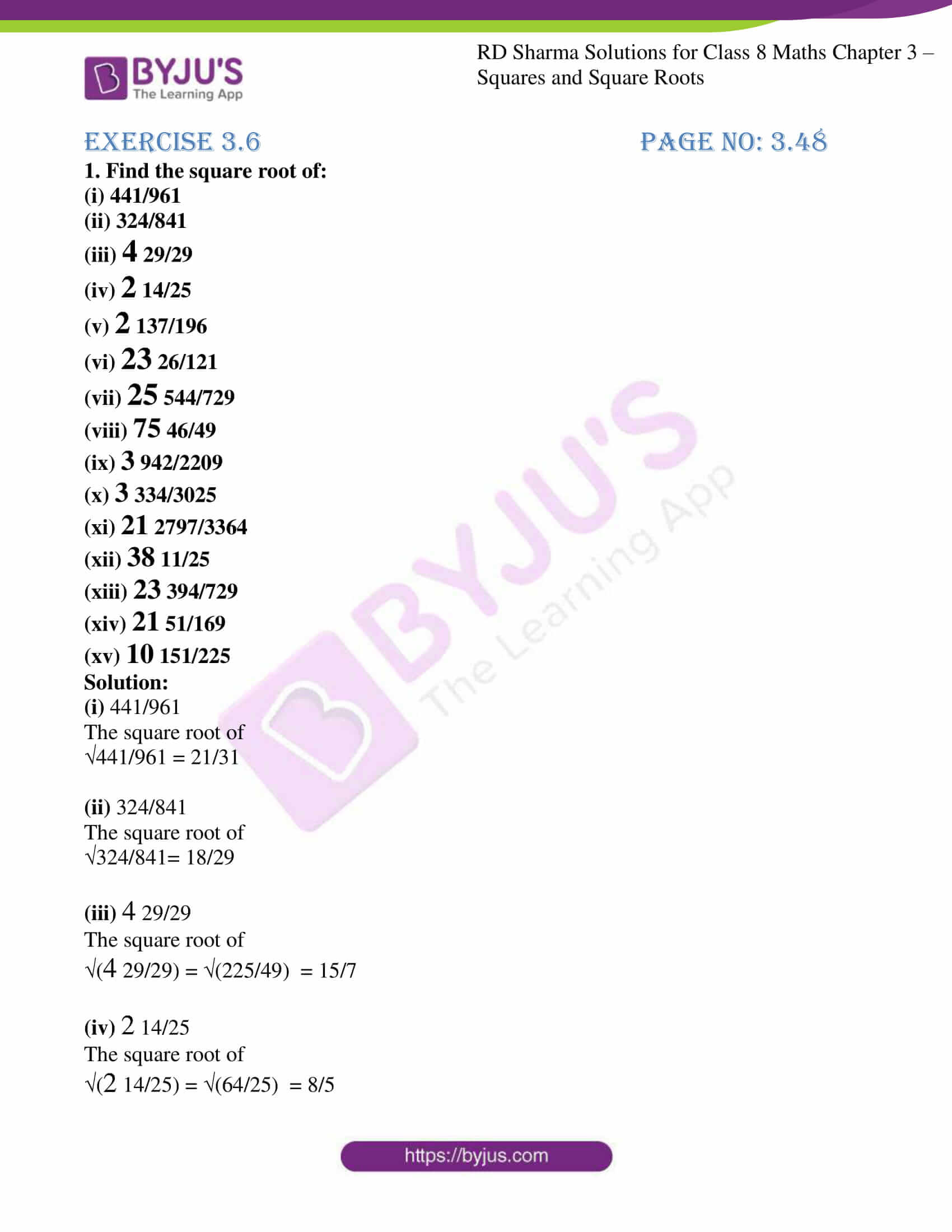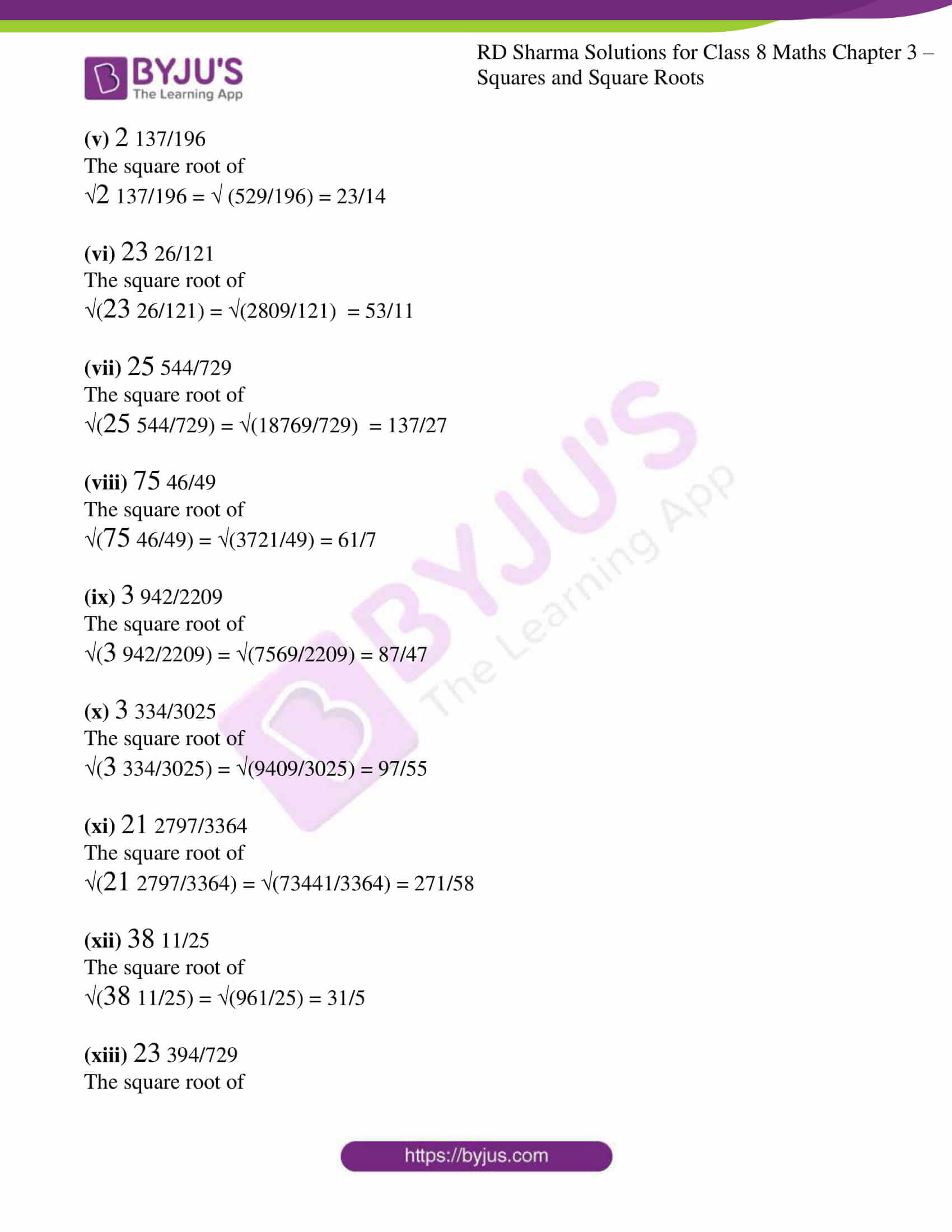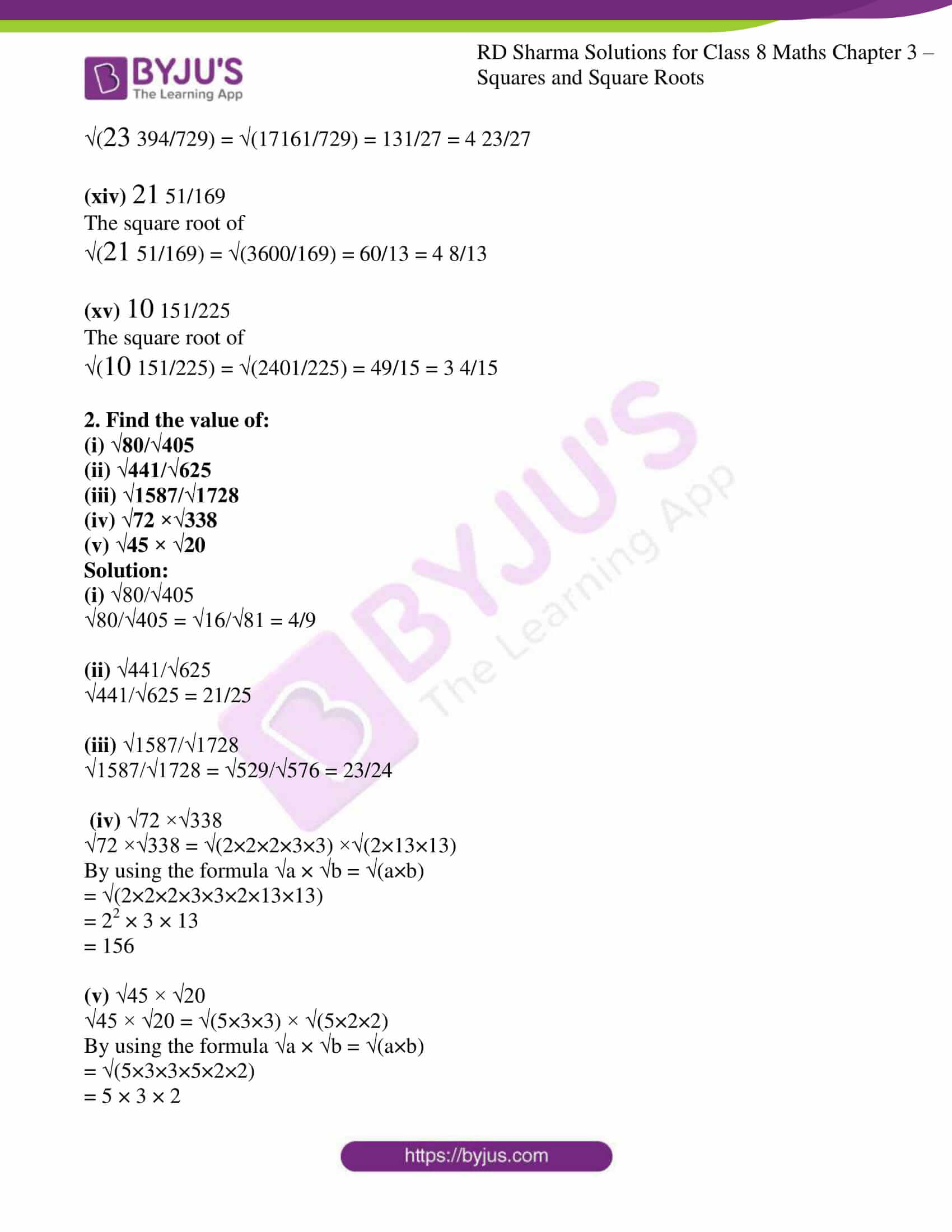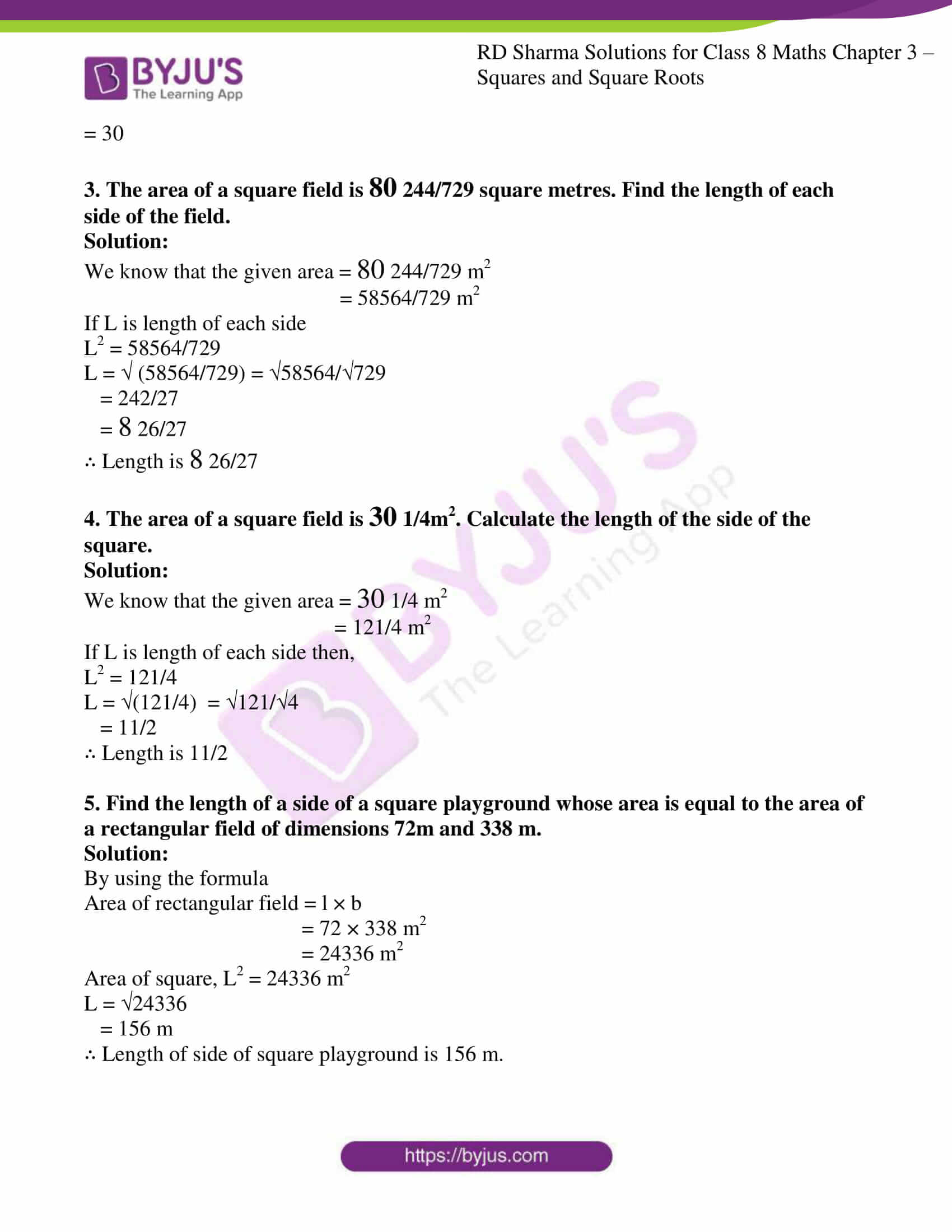# RD Sharma Solutions for Class 8 Maths Chapter 3 - Squares and Square Roots Exercise 3.6

Students can access the links given below to RD Sharma Solutions for Class 8 Maths Exercise 3.6 Chapter 3, Squares and Square Roots. The questions present in this exercise have been solved by BYJU’S experts in Maths, and this will help students in solving Exercise 3.6 of Class 8 without any hassle. Exercise 3.6 of Class 8 helps students to understand the concept of finding the square root of rational numbers in the form of fractions.

By practising the RD Sharma Solutions, Class 8, students gain confidence which plays a vital role in their examinations.

## Download the pdf of RD Sharma Solutions For Class 8 Maths Chapter 3 Squares and Square Roots Exercise 3.6### Access Answers to RD Sharma Solutions for Class 8 Maths Exercise 3.6 Chapter 3 Squares and Square Roots

#### EXERCISE 3.6 PAGE NO: 3.48

1. Find the square root of:

(i) 441/961

(ii) 324/841

(iii) 4 29/29

(iv) 2 14/25

(v) 2 137/196

(vi) 23 26/121

(vii) 25 544/729

(viii) 75 46/49

(ix) 3 942/2209

(x) 3 334/3025

(xi) 21 2797/3364

(xii) 38 11/25

(xiii) 23 394/729

(xiv) 21 51/169

(xv) 10 151/225

Solution:

(i) 441/961

The square root of

√441/961 = 21/31

(ii) 324/841

The square root of

√324/841= 18/29

(iii) 4 29/29

The square root of

√(4 29/29) = √(225/49) = 15/7

(iv) 2 14/25

The square root of

√(2 14/25) = √(64/25) = 8/5

(v) 2 137/196

The square root of

√2 137/196 = √ (529/196) = 23/14

(vi) 23 26/121

The square root of

√(23 26/121) = √(2809/121) = 53/11

(vii) 25 544/729

The square root of

√(25 544/729) = √(18769/729) = 137/27

(viii) 75 46/49

The square root of

√(75 46/49) = √(3721/49) = 61/7

(ix) 3 942/2209

The square root of

√(3 942/2209) = √(7569/2209) = 87/47

(x) 3 334/3025

The square root of

√(3 334/3025) = √(9409/3025) = 97/55

(xi) 21 2797/3364

The square root of

√(21 2797/3364) = √(73441/3364) = 271/58

(xii) 38 11/25

The square root of

√(38 11/25) = √(961/25) = 31/5

(xiii) 23 394/729

The square root of

√(23 394/729) = √(17161/729) = 131/27 = 4 23/27

(xiv) 21 51/169

The square root of

√(21 51/169) = √(3600/169) = 60/13 = 4 8/13

(xv) 10 151/225

The square root of

√(10 151/225) = √(2401/225) = 49/15 = 3 4/15

2. Find the value of:

(i) √80/√405

(ii) √441/√625

(iii) √1587/√1728

(iv) √72 ×√338

(v) √45 × √20

Solution:

(i) √80/√405

√80/√405 = √16/√81 = 4/9

(ii) √441/√625

√441/√625 = 21/25

(iii) √1587/√1728

√1587/√1728 = √529/√576 = 23/24

(iv) √72 ×√338

√72 ×√338 = √(2×2×2×3×3) ×√(2×13×13)

By using the formula √a × √b = √(a×b)

= √(2×2×2×3×3×2×13×13)

= 22 × 3 × 13

= 156

(v) √45 × √20

√45 × √20 = √(5×3×3) × √(5×2×2)

By using the formula √a × √b = √(a×b)

= √(5×3×3×5×2×2)

= 5 × 3 × 2

= 30

3. The area of a square field is 80 244/729 square metres. Find the length of each side of the field.

Solution:

We know that the given area = 80 244/729 m2

= 58564/729 m2

If L is length of each side

L2 = 58564/729

L = √ (58564/729) = √58564/√729

= 242/27

= 8 26/27

∴ Length is 8 26/27

4. The area of a square field is 30 1/4m2. Calculate the length of the side of the square.

Solution:

We know that the given area = 30 1/4 m2

= 121/4 m2

If L is length of each side then,

L2 = 121/4

L = √(121/4)  = √121/√4

= 11/2

∴ Length is 11/2

5. Find the length of a side of a square playground whose area is equal to the area of a rectangular field of dimensions 72m and 338 m.

Solution:

By using the formula

Area of rectangular field = l × b

= 72 × 338 m2

= 24336 m2

Area of square, L2 = 24336 m2

L = √24336

= 156 m

∴ Length of side of square playground is 156 m.

## RD Sharma Solutions for Class 8 Maths Exercise 3.6 Chapter 3 – Squares and Square Roots

Exercise 3.6 of RD Sharma Solutions for Chapter 3 Squares and Square Roots, this exercise mainly deals with the concepts related to the square roots of rational numbers in the form of fractions.
RD Sharma Class 8 Solutions can help the students in practising and learning each and every concept as it provides solutions to all questions asked in the RD Sharma textbook. Those who aim to secure high marks in Maths of Class 8 are advised to practice all the questions present in the textbook. Very lengthy questions that require many steps can also be easily remembered by students by using RD Sharma Solutions.